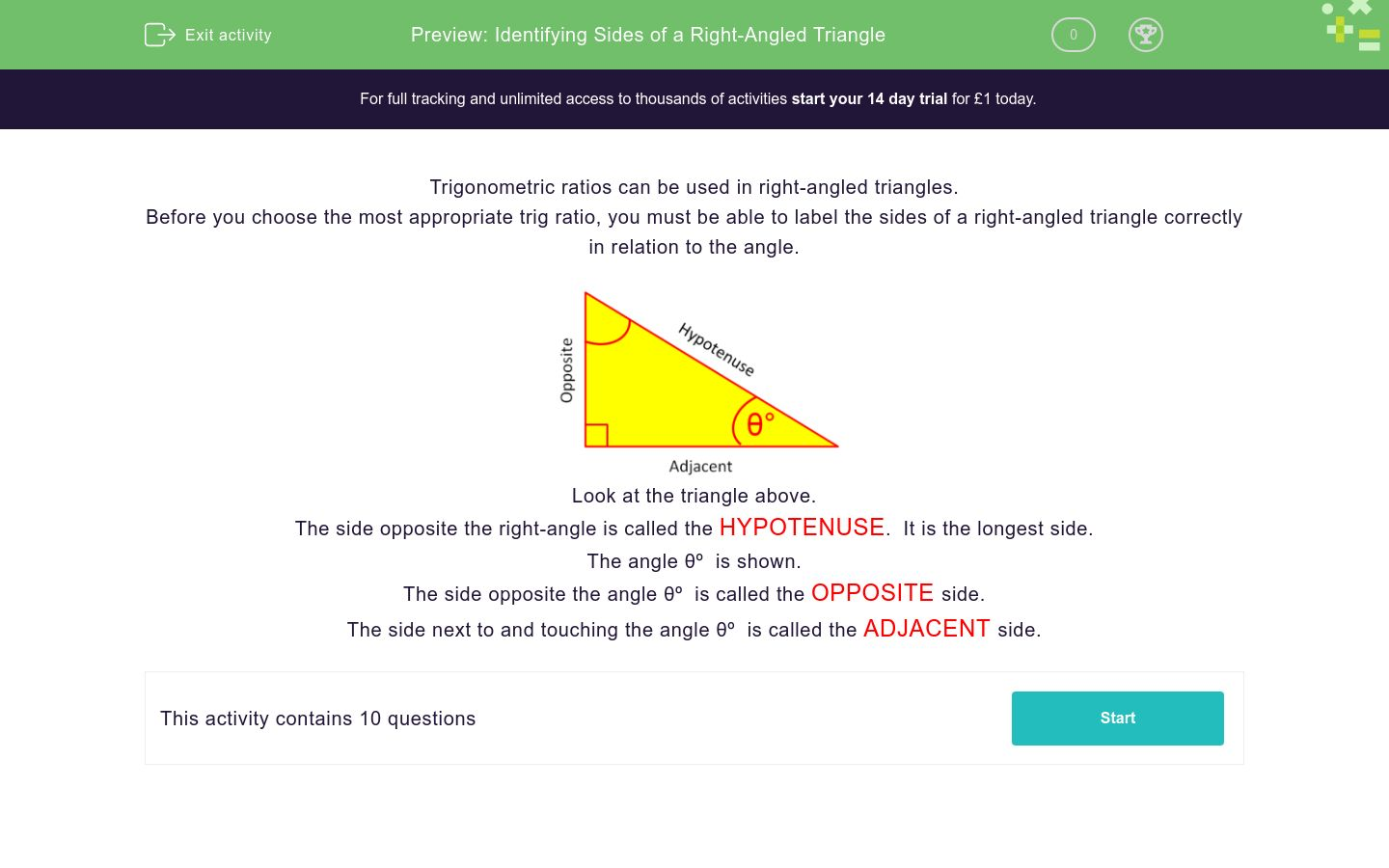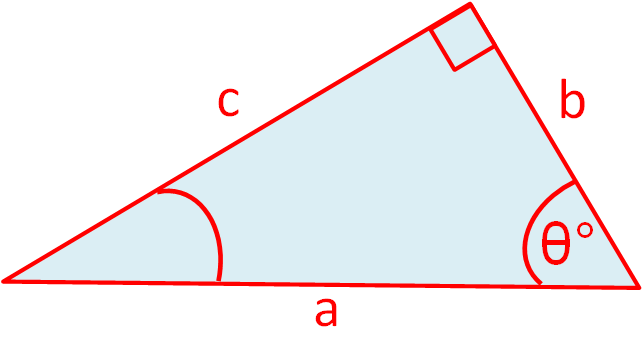# Identifying Sides of a Right-Angled Triangle

In this worksheet, students label the sides of right-angled triangles as adjacent, opposite or hypotenuse.Key stage:  KS 3

Curriculum topic:   Geometry and Measures

Curriculum subtopic:   Solve Right-Angled Triangle Problems

Difficulty level:### QUESTION 1 of 10

Trigonometric ratios can be used in right-angled triangles.

Before you choose the most appropriate trig ratio, you must be able to label the sides of a right-angled triangle correctly in relation to the angle.Look at the triangle above.

The side opposite the right-angle is called the HYPOTENUSE.  It is the longest side.

The angle θº  is shown.

The side opposite the angle θº  is called the OPPOSITE side.

The side next to and touching the angle θº  is called the ADJACENT side.

In relation to the angle θ, label the side marked a in this right-angled triangle.Opposite

Hypotenuse

In relation to the angle θ, label the side marked b in this right-angled triangle.Opposite

Hypotenuse

In relation to the angle θ, label the side marked c in this right-angled triangle.Opposite

Hypotenuse

In relation to the angle θ, label the side marked c in this right-angled triangle.Opposite

Hypotenuse

In relation to the angle θ, label the side marked b in this right-angled triangle.Opposite

Hypotenuse

In relation to the angle θ, label the side marked a in this right-angled triangle.Opposite

Hypotenuse

In relation to the angle θ, label the side marked a in this right-angled triangle.Opposite

Hypotenuse

In relation to the angle θ, label the side marked b in this right-angled triangle.Opposite

Hypotenuse

In relation to the angle θ, label the side marked b in this right-angled triangle.Opposite

Hypotenuse

In relation to the angle θ, label the side marked a in this right-angled triangle.Opposite

Hypotenuse

• Question 1

In relation to the angle θ, label the side marked a in this right-angled triangle.Opposite
EDDIE SAYS
a is opposite the angle given.
• Question 2

In relation to the angle θ, label the side marked b in this right-angled triangle.EDDIE SAYS
b is adjacent to the angle given and is not opposite the right angle
• Question 3

In relation to the angle θ, label the side marked c in this right-angled triangle.Hypotenuse
EDDIE SAYS
c is opposite the right angle.
• Question 4

In relation to the angle θ, label the side marked c in this right-angled triangle.Opposite
EDDIE SAYS
c is adjacent to the angle given and is not opposite the right angle
• Question 5

In relation to the angle θ, label the side marked b in this right-angled triangle.Hypotenuse
EDDIE SAYS
b is adjacent to the right angle
• Question 6

In relation to the angle θ, label the side marked a in this right-angled triangle.EDDIE SAYS
a is apposite the angle given
• Question 7

In relation to the angle θ, label the side marked a in this right-angled triangle.Hypotenuse
EDDIE SAYS
b is opposite the right angle
• Question 8

In relation to the angle θ, label the side marked b in this right-angled triangle.EDDIE SAYS
b is adjacent to the angle given and is not opposite the right angle
• Question 9

In relation to the angle θ, label the side marked b in this right-angled triangle.Hypotenuse
EDDIE SAYS
b is opposite the right angle
• Question 10

In relation to the angle θ, label the side marked a in this right-angled triangle.Opposite
EDDIE SAYS
a is opposite the angle given.
---- OR ----

Sign up for a £1 trial so you can track and measure your child's progress on this activity.

### What is EdPlace?

We're your National Curriculum aligned online education content provider helping each child succeed in English, maths and science from year 1 to GCSE. With an EdPlace account you’ll be able to track and measure progress, helping each child achieve their best. We build confidence and attainment by personalising each child’s learning at a level that suits them.

Get started